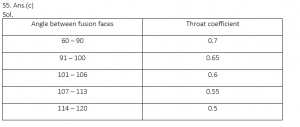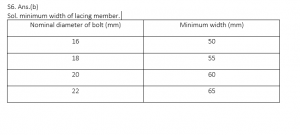Engineering Jobs   »   CIVIL ENGINEERING QUIZ

# DFCCIL’21 CE: Daily Practice Quiz. 22-July-2021

Quiz: Civil Engineering
Exam: DFCCIL-JE
Topic: MISCELLANEOUS

Each question carries 1 mark
Negative marking: 1/4 mark
Time: 8 Minutes

Q1. In Versenate method (Hardness test) the water is titrated against EDTA salt solution using Eriochrome Black T as indicator solution. Here at the end titration, the color changes from
(a) Wine red to blue
(b) Blue to sea green
(c) Yellow to blue
(d) Blue to yellow

Q2. The pH value of the incoming water is 7.2 and outgoing water is 8.4, assuming a linear variation of pH with time determine average pH value of water:
(a) 7.800
(b) 7.474
(c) 7.2
(d) 8.4

Q3. Hydrometer is used to determine-
(a) density of liquids
(b) flow of liquids
(c) relative humidity
(d) specific gravity of the liquid

Q4. IRC recommendation for maximum width of a vehicle is
(a) 1.75 m
(b) 2.00 m
(c) 2.44 m
(d) 4.88 m

Q5. Fillet weld is not recommending if the angle between fusion faces is ___________:
A. Less than 45°
B. Greater than 120°
C. Less than 60°
D. Greater than 145°
The correct statement are
(a) A&B
(b) A&D
(c) B&C
(d) C&D

Q6. If the 20 mm rivets are used in lacing bars, then the minimum width of lacing bar, should be
(a) 40 mm
(b) 60 mm
(c) 80 mm
(d) 100 mm

SOLUTION

S1. Ans.(a)
Sol. in versenate method (hardness test), when the water is titrated against EDTA salt solution using Eriochrome Black T as indicator, Then the color of solution changes from wine red to blue.

S2. Ans.(b)
Sol. pH of incoming water = 7.2
pH of outgoing water = 8.4
Average pH value of water =?
pH = – log [H+]
7.2 = – log [H+]
Tanking anti log-
[H+] = 10^(-7.2)———— (1)
pH = – log [H+]
8.4 = – log [H+]
Tanking anti log-
[H+] = 10^(-8.4)———— (2)
Average concentration of [H+] = (10^(-7.2)+10^(-8.4))/2

Average pH = – log (10^(-7.2)+10^(-8.4))/2
= – log [3.35384×10^(-8) ]
= -(-7.47445762)
= 7.474
S3. Ans.(d)
Sol. Hydrometer = it is an instrument which is used to measure the density of liquid with reference of water i.e. specific gravity.
S4. Ans.(c)
Sol. As per IRC
(a) Maximum width of vehicle = 2.44 m.
(b) Maximum height of vehicle = 4.75 m.
(c) Maximum length of vehicle = 18 m.
(d) Maximum length of vehicle = 52.2 Tonn.Sharing is caring!